Courses

# Hydrodynamics (Part - 1) - Mechanics, Irodov JEE Notes | EduRev

## NEET : Hydrodynamics (Part - 1) - Mechanics, Irodov JEE Notes | EduRev

The document Hydrodynamics (Part - 1) - Mechanics, Irodov JEE Notes | EduRev is a part of the NEET Course Physics For JEE.
All you need of NEET at this link: NEET

Q. 315. Ideal fluid flows along a flat tube of constant cross-section, located in a horizontal plane and bent as shown in Fig. 1.80 (top view). The flow is steady. Are the pressures and velocities of the fluid equal at points 1 and 2? What is the shape of the streamlines?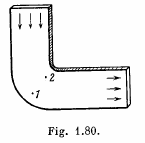Solution. 315. Between 1 and 2 fluid particles are in nearly circular motion and therefore have centripetal acceleration. The force for this acceleration, like for any other situation in an ideal fluid, can only come from the pressure variation along the line joining 1 and 2. This requires that pressure at 1 should be greater than the pressure at 2 i.e.

P1 > P2

so that the fluid particles can have required acceleration. If there is no turbulence, the motion can be taken as irrotational. Then by considering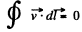along the circuit shown we infer that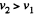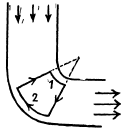(The portion of the circuit near 1 and 2 are streamlines while the other two arms are at right angle to streamlines)

In an incompressible liquid we also have div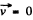By electrostatic analogy we then find that the density of streamlines is proportional to the velocity at that point.

Q. 316. Two manometric tubes are mounted on a horizontal pipe of varying cross-section at the sections S1 and S2 (Fig. 1.81). Find the volume of water flowing across the pipe's section per unit time if the difference in water columns is equal to Δh.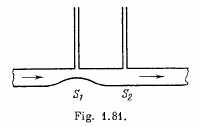Solution. 316. From the conservation of mass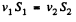(1)

But  S1 < S2 as shown in the figure of the problem, therefore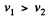As every streamline is horizontal between 1 & 2, Bemoull’s theorem becomes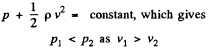As the difference in height o f the water column is Δh , therefore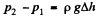(2)

From Bernoulli theorem between points 1 and 2 of a streamline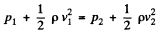or,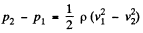or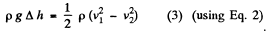using (1) in (3), we get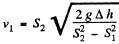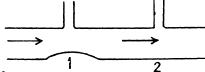Hence the sought volume of water flowing per see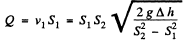Q. 317. A Pitot tube (Fig. 1.82) is mounted along the axis of a gas pipeline whose cross-sectional area is equal to S. Assuming the viscosity to be negligible, find the volume of gas flowing across the section of the pipe per unit time, if the difference in the liquid columns is equal to Δh, and the densities of the liquid and the gas are P0 and p respectively;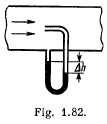Solution. 317. Applying Bernoulli’s theorem for the point A and B,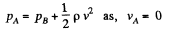or,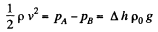So,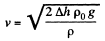Thus, rate of flow of gas,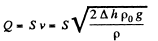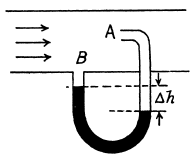The gas flows over the tube past it at B. But at A the gas becomes stationary as the gas will move into the tube which already contains gas.

In applying Bernoulli’s theorem we should remember that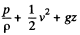is constant along a streamline. In the present case, we are really applying Bernoulli's theorem somewhat indirectly. The streamline at A is not the streamline at B. Nevertheless the result is correct. To be convinced of this, we need only apply Bernoulli theorem to the streamline that goes through A by comparing the situation at A with that above B on the same level. In steady conditions, this agrees with the result derived because there cannot be a transverse pressure differential.

Q. 318. A wide vessel with a small hole in the bottom is filled with water and kerosene. Neglecting the viscosity, find the velocity of the water flow, if the thickness of the water layer is equal to h1 = 30 cm and that of the kerosene layer to h2  = 20 cm.

Solution. 318. Since, the density of water is greater than that of kerosene oil, it will collect at the bottom. Now, pressure due to water level equals h1 p1 g and pressure due to kerosene oil level equals h2 p2 g. So, net pressure becomes h1 p1 g + h2 p2 g.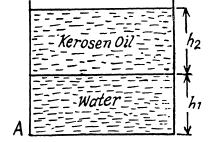From Bernoulli’s theorem, this pressure energy will be converted into kinetic energy while flowing through the whole A.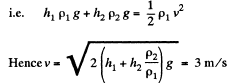Q. 319. A wide cylindrical vessel 50 cm in height is filled with water and rests on a table. Assuming the viscosity to be negligible, find at what height from the bottom of the vessel a small hole should be perforated for the water jet coming out of it to hit the surface of the table at the maximum distance lmax  from the vessel. Find lmax.

Solution. 319. Let, H be the total height of water column and the hole is made at a height h from the bottom.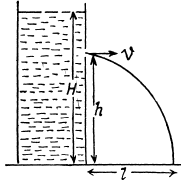Then from Bernoulli’s theorem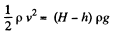or,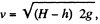which is directed h orizontally.

For the horizontal range, l = v t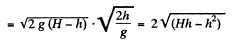Now, for maximum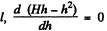which yields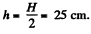Q. 320. A bent tube is lowered into a water stream as shown in Fig. 1.83. The velocity of the stream relative to the tube is equal to v = 2.5 m/s. The closed upper end of the tube located at the height 12, h0 = 12 cm has a small orifice. To what height h will the water jet spurt?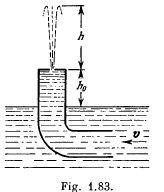Solution. 320. Let the velocity of the water jet, near the orifice be v', then applying Bemoullis theorem,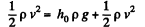or,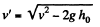(1)

Here the pressure term on both sides is the same and equal to atmospheric pressure. (In the problem book Fig. should be more clear.)

Now, if it rises upto a height h, then at this height, whole of its kinetic energy will be converted into potential energy. So,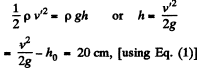Q. 321. The horizontal bottom of a wide vessel with an ideal fluid has a round orifice of radius R1 over which a round closed cylinder is mounted, whose radius R2 > R1 ( Fig. 1.84). The clearance between the cylinder and the bottom of the vessel is very small, the fluid density is p. Find the static pressure of the fluid in the clearance as a function of the distance r from the axis of the orifice (and the cylinder), if the height of the fluid is equal to h.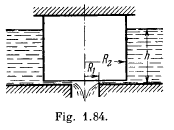Solution. 321. Water flows through the small clearance into the orifice. Let d be the clearance. Then from the equation of continuity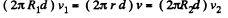or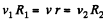(1)

where v1, v2 and v are respectively the inward radial velocities of the fluid at 1, 2 and 3.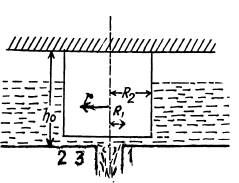Now by Bernoulli's theorem just before 2 and just after it in the clearance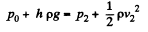(2)

Applying the same theorem at 3 and 1 we find that this also equals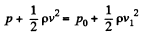(3)

(since the pressure in the orifice is p0)

From Eqs. (2) and (3) we also hence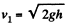(4)

and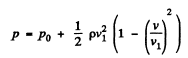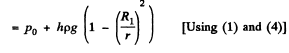Q. 322. What work should be done in order to squeeze all water from a horizontally located cylinder (Fig. 1.85) during the time t by means of a constant force acting on the piston? The volume of water in the cylinder is equal to V, the cross-sectional area of the ori- fice to s, with s being considerably less than the piston area. The friction and viscosity are negligibly small.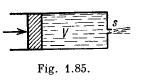Solution. 322. Let the force acting on the piston he F and foe length oi the cylinder be l.

Then, work done = FI (1)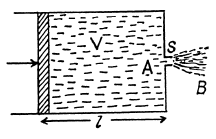Applying Bernoulli's theorem for points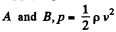where P is density and v is the velocity at point B. Now, force on the piston,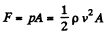(2)

where A is the cross section area of piston.
Also, discharge through the orifice during time interval t = Svt and this is equal to the volume of the cylinder, i.e.,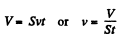(3)

From Eq. (1), (2) and (3) work done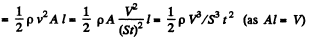Q. 323. A cylindrical vessel of height h and base area S is filled with water. An orifice of area s ≪ S is opened in the bottom of the vessel. Neglecting the  viscosity of water, determine how soon all the water will pour out of the vessel.

Solution. 323. Let at any moment of time, water level in the vessel be H then speed of' flow of water through the orifice, at that moment will be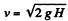(1)

In the time interval dt, the volume of water ejected through orifice,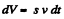(2)

On the other hand, the volume of water in the vessel at time t equals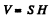Differentiating (3) with respect to time,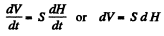(4)

Eqs. (2) and (4)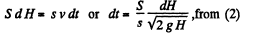Integrating,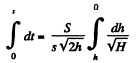Thus,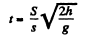Q. 324. A  horizontally  oriented  tube AB of length 1 rotates with a constant angular velocity ω about a stationary vertical axis OO' passing through the end A (Fig. 1.86). The tube is filled with an ideal fluid. The  end  A of the tube is open the closed end B has a very small orifice. Find the velocity of the fluid relative to the  tube  as  a  function of the column "height" h.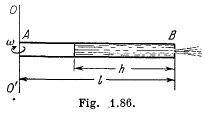Solution. 324. In a rotating frame (with constant angular velocity) the Eulerian equation is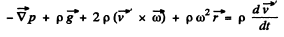In the frame of rotating tube the liquid in the "column* is practically static because the orifice is sufficiently small. Thus the Eulerian Eq. in projection form along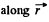(which is the position vector of an arbitrary liquid element of lenth dr relative to the rotation axis reduces to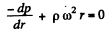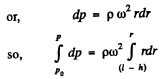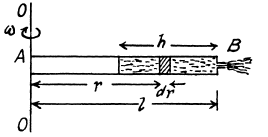Thus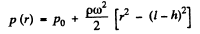(1)

Hence the pressure at the end B just before the orifice i.e.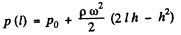(2)

Then applying Bemouirs theorem at the orifice for the points just inside and outside of the end B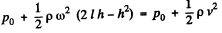(where v is the sought velocity)

So,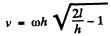Q. 325. Demonstrate that in the case of a steady flow of an ideal fluid Eq. (1.7a) turns into Bernoulli equation.

Solution. 325.  The Euler’s equation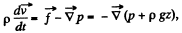where z is vprtirallv upwards.

Now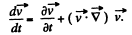(1)

But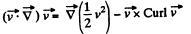(2)

we consider the stead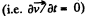flow of an incompressible fluid then p = constant.

and as the motion is irrotational Curl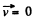So from (1) and (2)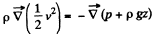or,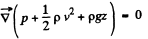Hence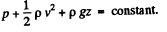Q. 326. On the opposite sides of a wide vertical vessel filled with water two identical holes are opened, each having the cross-sectional area S = 0.50 cm2. The height difference between them is equal to Δh = 51 cm. Find the resultant force of reaction of the water flowing out of the vessel.

Solution. 326. Let the velocity of water, flowing through A be vA and that through B be vB, then discharging rate through A = QA = S vA and similarly through B = S vB.

Now, force of reaction at A,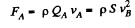Hence, the net force,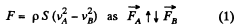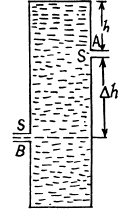Applying Bernoulli’s theorem to the liquid flowing out of A we get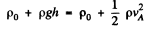and similarly at B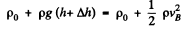Hence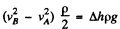Thus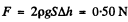Q. 327. The side wall of a wide vertical cylindrical vessel of height h = 75 cm has a narrow vertical slit running all the way down to the bottom of the vessel. The length of the slit is l = 50 cm and the width b = 1.0 mm. With the slit closed, the vessel is filled with water. Find the resultant force of reaction of the water flowing out of the vessel immediately after the slit is opened.

Solution. 327. Consider an element of height dy at a distance y from the top. The velocity of the fluid coming out of the element is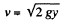The force of reaction dF due to this is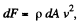as in the previous problem,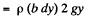Integrating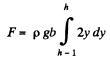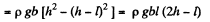(The slit runs from a depth h - l to a depth h from the top.)

Offer running on EduRev: Apply code STAYHOME200 to get INR 200 off on our premium plan EduRev Infinity!

## Physics For JEE

187 videos|516 docs|263 tests

,

,

,

,

,

,

,

,

,

,

,

,

,

,

,

,

,

,

,

,

,

,

,

,

;